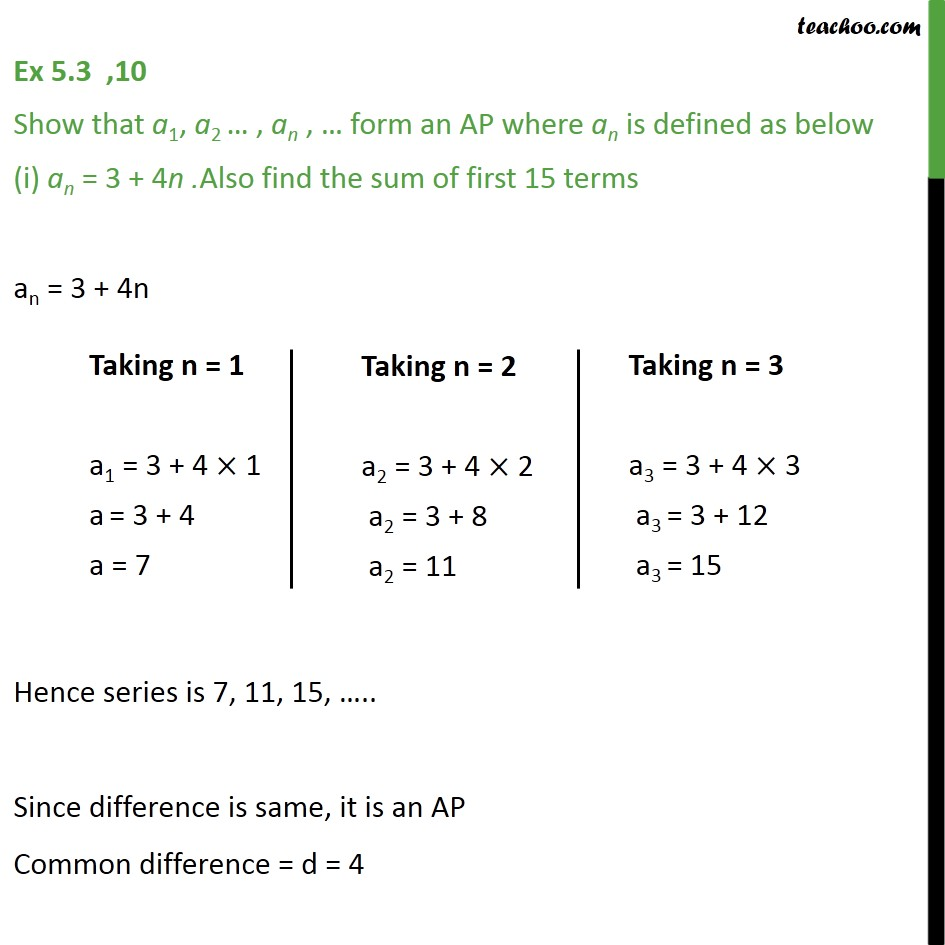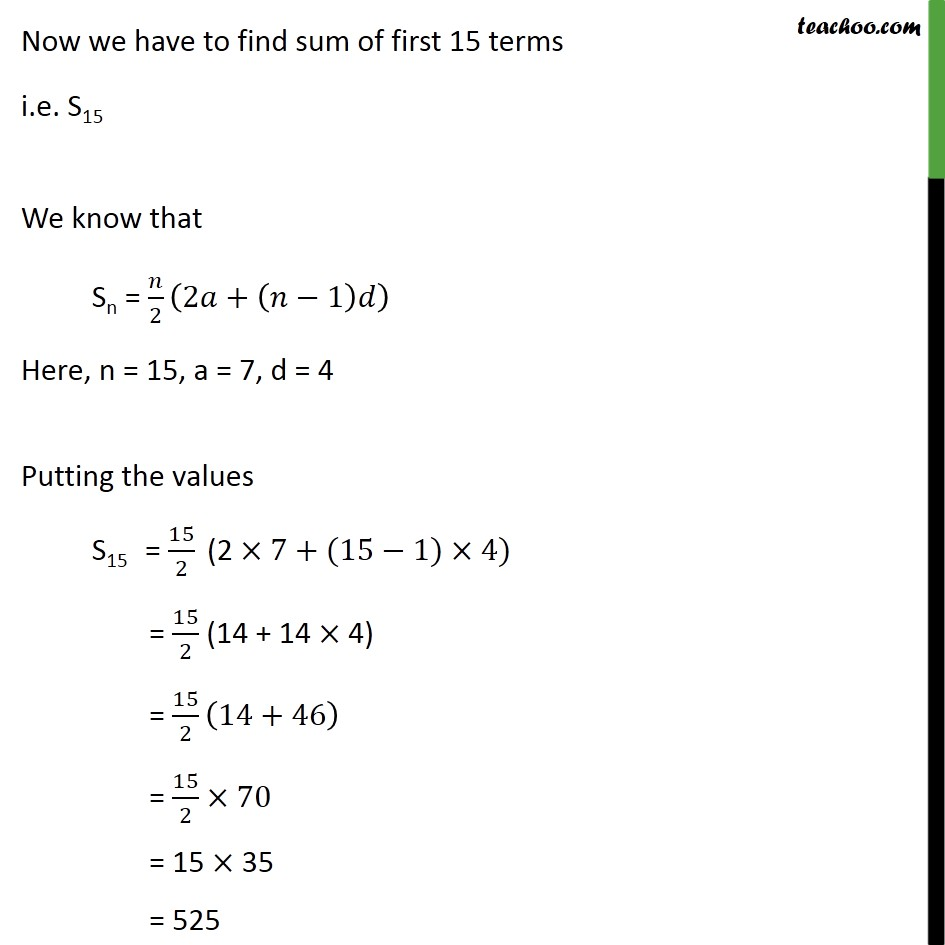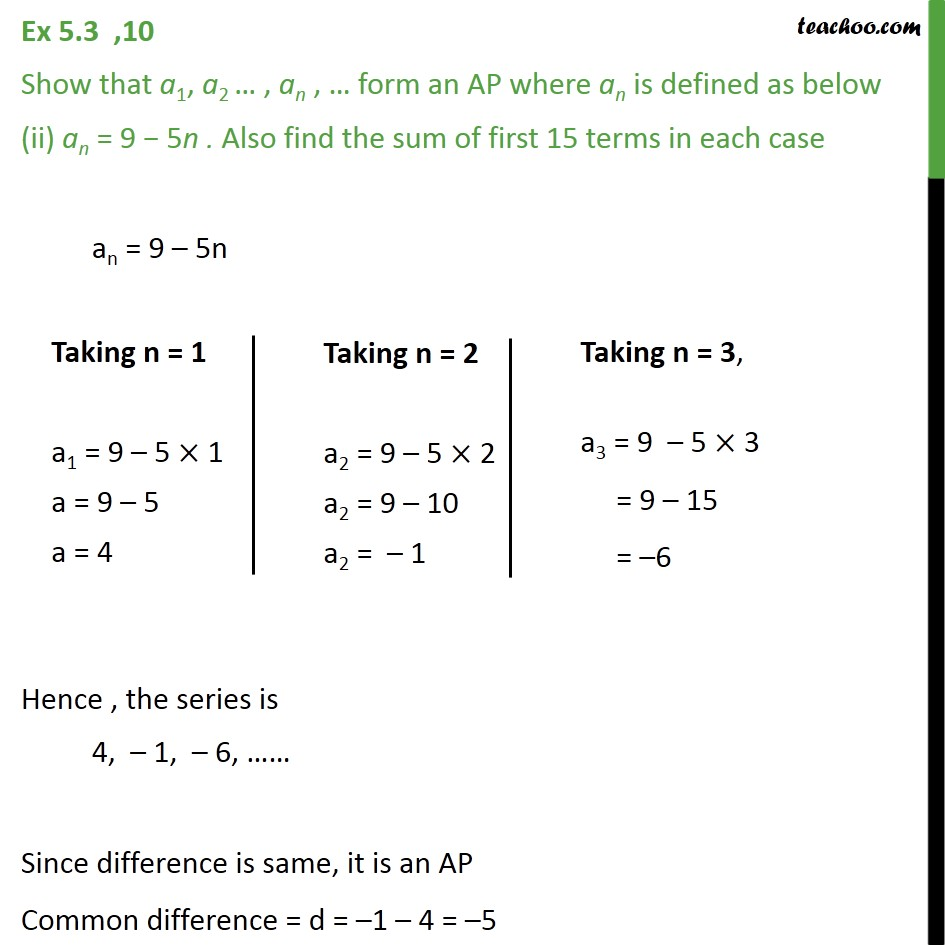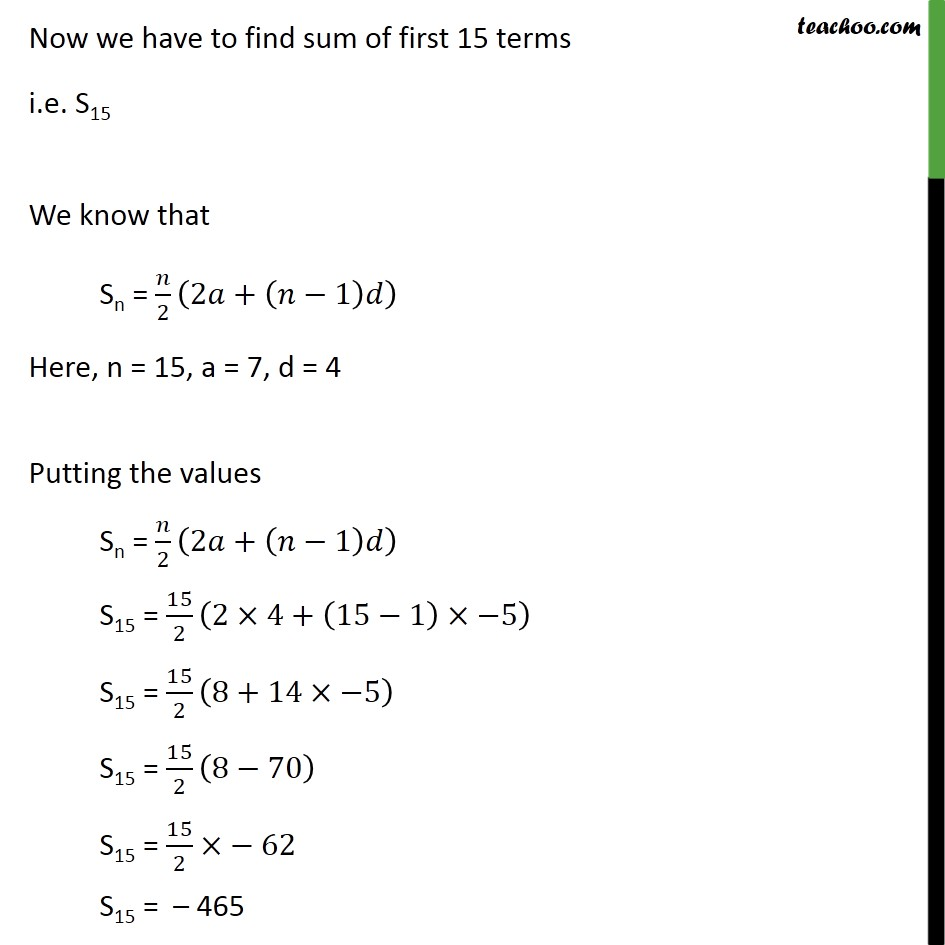Learn all Concepts of Chapter 5 Class 10 (with VIDEOS). Check - Arithmetic Progressions - Class 101. Chapter 5 Class 10 Arithmetic Progressions
2. Serial order wise
3. Ex 5.3

Transcript

Ex 5.3 ,10 Show that a1, a2 , an , form an AP where an is defined as below (i) an = 3 + 4n .Also find the sum of first 15 terms an = 3 + 4n Hence series is 7, 11, 15, .. Since difference is same, it is an AP Common difference = d = 4 Now we have to find sum of first 15 terms i.e. S15 We know that Sn = /2 (2 +( 1) ) Here, n = 15, a = 7, d = 4 Putting the values S15 = 15/2 (2 7+(15 1) 4) = 15/2 (14 + 14 4) = 15/2 (14+46) = 15/2 70 = 15 35 = 525 Ex 5.3 ,10 Show that a1, a2 , an , form an AP where an is defined as below (ii) an = 9 5n . Also find the sum of first 15 terms in each case an = 9 5n Hence , the series is 4, 1, 6, Since difference is same, it is an AP Common difference = d = 1 4 = 5 Now we have to find sum of first 15 terms i.e. S15 We know that Sn = /2 (2 +( 1) ) Here, n = 15, a = 7, d = 4 Putting the values Sn = /2 (2 +( 1) ) S15 = 15/2 (2 4+(15 1) 5) S15 = 15/2 (8+14 5) S15 = 15/2 (8 70) S15 = 15/2 62 S15 = 465

Ex 5.3